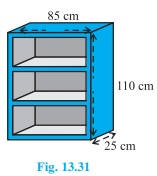# A wooden bookshelf has external dimensions as follows: Height = 110cm, Depth = 25cm, Breadth = 85cm (see fig) . The thickness of the plank is 5cm everywhere. The external faces are to be polished and the inner faces are to be painted. If the rate of polishing is 20 paise per cm2 and the rate of painting is 10 paise per cm2, find the total expenses required for polishing and painting the surface of the bookshelf.Given

Length of bookshelf (l) = 85cm

Breadth of bookshelf (b) = 25 cm

Height of bookshelf (h) = 110cm

Find out

We have to find out the total expenses required for polishing and painting the surface of the bookshelf.

Solution

To calculate the external surface area of the shelf while leaving out the front face of the shelf = lh+2(lb+bh)

= [85×110+2(85×25+25×110)]

= (9350+9750)

= 19100 cm2

Hence, the external surface area of shelf is 19100 cm2

Area of front face = [85×110-75×100+2(75×5)]

= 1850+750

= 2600 cm2

Thus, the area is 2600 cm2

Area to be polished surface = (19100+2600) cm2

= 21700 cm2

Given cost of polishing 1 cm2 area = Rs 0.20

Therefore, the cost of polishing 21700 cm2 area Rs. (21700×0.20)

= Rs 4340

Dimensions of row of the book shelf

Length(l) = 75 cm

Breadth (b) = 20 cm and

Height(h) = 30 cm

Area to be painted in one row= 2(l+h)b+lh

= [2(75+30)× 20+75×30]

= (4200+2250)

= 6450

So, area is 6450 cm2.

Painting area

Area to be painted in 3 rows = (3×6450)cm= 19350 cm2.

Cost of painting 1 cm2 area = Rs. 0.10

Cost of painting 19350 cm2 area = Rs (19350 x 0.1) = Rs 1935

Total expense required for polishing and painting = Rs. (4340+1935) = Rs. 6275

The cost for polishing and painting the surface of the bookshelf is Rs. 6275.